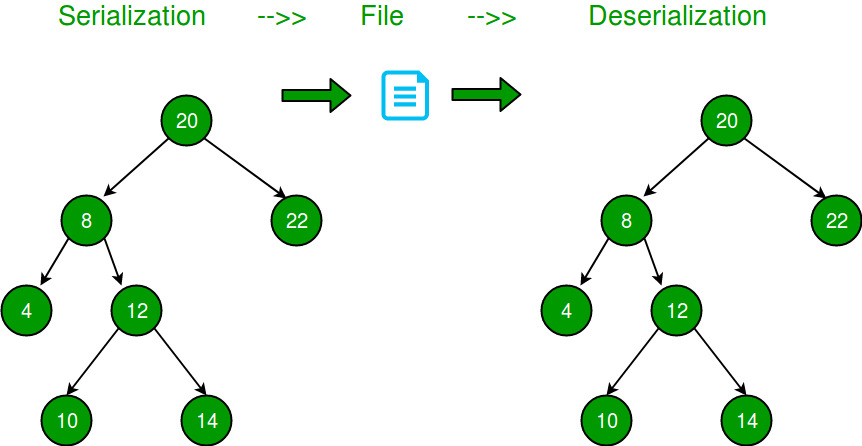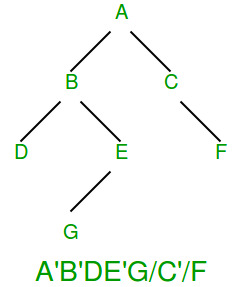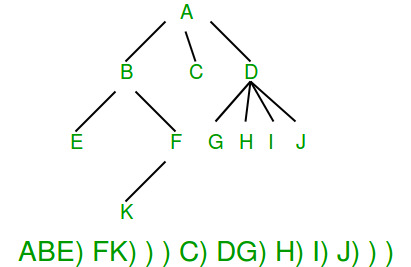# Serialize and Deserialize a Binary Tree

• Difficulty Level : Hard
• Last Updated : 23 Jun, 2022

Serialization is to store tree in a file so that it can be later restored. The structure of tree must be maintained. Deserialization is reading tree back from file.Following are some simpler versions of the problem:

If given Tree is Binary Search Tree?
If the given Binary Tree is Binary Search Tree, we can store it by either storing preorder or postorder traversal. In case of Binary Search Trees, only preorder or postorder traversal is sufficient to store structure information

If given Binary Tree is Complete Tree?
A Binary Tree is complete if all levels are completely filled except possibly the last level and all nodes of last level are as left as possible (Binary Heaps are complete Binary Tree). For a complete Binary Tree, level order traversal is sufficient to store the tree. We know that the first node is root, next two nodes are nodes of next level, next four nodes are nodes of 2nd level and so on.

If given Binary Tree is Full Tree?
A full Binary is a Binary Tree where every node has either 0 or 2 children. It is easy to serialize such trees as every internal node has 2 children. We can simply store preorder traversal and store a bit with every node to indicate whether the node is an internal node or a leaf node.

How to store a general Binary Tree?
A simple solution is to store both Inorder and Preorder traversals. This solution requires space twice the size of Binary Tree.
We can save space by storing Preorder traversal and a marker for NULL pointers.

```Let the marker for NULL pointers be '-1'
Input:
12
/
13
Output: 12 13 -1 -1 -1

Input:
20
/   \
8     22
Output: 20 8 -1 -1 22 -1 -1

Input:
20
/
8
/ \
4  12
/  \
10  14
Output: 20 8 4 -1 -1 12 10 -1 -1 14 -1 -1 -1

Input:
20
/
8
/
10
/
5
Output: 20 8 10 5 -1 -1 -1 -1 -1

Input:
20
\
8
\
10
\
5
Output: 20 -1 8 -1 10 -1 5 -1 -1```

Deserialization can be done by simply reading data from file one by one.

Following is the implementation of the above idea.

## C++

 `// A C++ program to demonstrate serialization and deserialization of` `// Binary Tree` `#include ` `#define MARKER -1`   `/* A binary tree Node has key, pointer to left and right children */` `struct` `Node` `{` `    ``int` `key;` `    ``struct` `Node* left, *right;` `};`   `/* Helper function that allocates a new Node with the` `   ``given key and NULL left and right pointers. */` `struct` `Node* newNode(``int` `key)` `{` `    ``struct` `Node* temp = ``new` `Node();` `    ``temp->key = key;` `    ``temp->left = temp->right = NULL;` `    ``return` `(temp);` `}`   `// This function stores a tree in a file pointed by fp` `void` `serialize(Node *root, ``FILE` `*fp)` `{` `    ``// If current node is NULL, store marker` `    ``if` `(root == NULL)` `    ``{` `        ``fprintf``(fp, ``"%d "``, MARKER);` `        ``return``;` `    ``}`   `    ``// Else, store current node and recur for its children` `    ``fprintf``(fp, ``"%d "``, root->key);` `    ``serialize(root->left, fp);` `    ``serialize(root->right, fp);` `}`   `// This function constructs a tree from a file pointed by 'fp'` `void` `deSerialize(Node *&root, ``FILE` `*fp)` `{` `    ``// Read next item from file. If there are no more items or next` `    ``// item is marker, then return` `    ``int` `val;` `    ``if` `( !``fscanf``(fp, ``"%d "``, &val) || val == MARKER)` `       ``return``;`   `    ``// Else create node with this item and recur for children` `    ``root = newNode(val);` `    ``deSerialize(root->left, fp);` `    ``deSerialize(root->right, fp);` `}`   `// A simple inorder traversal used for testing the constructed tree` `void` `inorder(Node *root)` `{` `    ``if` `(root)` `    ``{` `        ``inorder(root->left);` `        ``printf``(``"%d "``, root->key);` `        ``inorder(root->right);` `    ``}` `}`   `/* Driver program to test above functions*/` `int` `main()` `{` `    ``// Let us construct a tree shown in the above figure` `    ``struct` `Node *root        = newNode(20);` `    ``root->left               = newNode(8);` `    ``root->right              = newNode(22);` `    ``root->left->left         = newNode(4);` `    ``root->left->right        = newNode(12);` `    ``root->left->right->left  = newNode(10);` `    ``root->left->right->right = newNode(14);`   `    ``// Let us open a file and serialize the tree into the file` `    ``FILE` `*fp = ``fopen``(``"tree.txt"``, ``"w"``);` `    ``if` `(fp == NULL)` `    ``{` `        ``puts``(``"Could not open file"``);` `        ``return` `0;` `    ``}` `    ``serialize(root, fp);` `    ``fclose``(fp);`   `    ``// Let us deserialize the stored tree into root1` `    ``Node *root1 = NULL;` `    ``fp = ``fopen``(``"tree.txt"``, ``"r"``);` `    ``deSerialize(root1, fp);`   `    ``printf``(``"Inorder Traversal of the tree constructed from file:\n"``);` `    ``inorder(root1);`   `    ``return` `0;` `}`

## Java

 `// A JAVA program to demonstrate serialization and` `// deserialization of Binary Tree` `import` `java.util.*;`   `/* A binary tree Node has key, pointer to left and right` ` ``* children */` `class` `TreeNode {` `    ``int` `val;` `    ``TreeNode left;` `    ``TreeNode right;` `    ``TreeNode(``int` `x) { val = x; }` `}`   `class` `BinaryTree {` `    ``TreeNode root;`   `    ``// Encodes a tree to a single string.` `    ``public` `static` `String serialize(TreeNode root)` `    ``{` `        ``if` `(root == ``null``) {` `            ``return` `null``;` `        ``}` `        ``Stack s = ``new` `Stack<>();` `        ``s.push(root);`   `        ``List l = ``new` `ArrayList<>();` `        ``while` `(!s.isEmpty()) {` `            ``TreeNode t = s.pop();` `            ``// If current node is NULL, store marker` `            ``if` `(t == ``null``) {` `                ``l.add(``"#"``);` `            ``}` `            ``else` `{` `                ``// Else, store current node and recur for` `                ``// its children` `                ``l.add(``""` `+ t.val);` `                ``s.push(t.right);` `                ``s.push(t.left);` `            ``}` `        ``}` `        ``return` `String.join(``","``, l);` `    ``}`   `    ``static` `int` `t;`   `    ``// Decodes your encoded data to tree.` `    ``public` `static` `TreeNode deserialize(String data)` `    ``{` `        ``if` `(data == ``null``)` `            ``return` `null``;` `        ``t = ``0``;` `        ``String[] arr = data.split(``","``);` `        ``return` `helper(arr);` `    ``}`   `    ``public` `static` `TreeNode helper(String[] arr)` `    ``{` `        ``if` `(arr[t].equals(``"#"``))` `            ``return` `null``;` `        ``// create node with this item and recur for children` `        ``TreeNode root` `            ``= ``new` `TreeNode(Integer.parseInt(arr[t]));` `        ``t++;` `        ``root.left = helper(arr);` `        ``t++;` `        ``root.right = helper(arr);` `        ``return` `root;` `    ``}`   `    ``// A simple inorder traversal used for testing the` `    ``// constructed tree` `    ``static` `void` `inorder(TreeNode root)` `    ``{` `        ``if` `(root != ``null``) {` `            ``inorder(root.left);` `            ``System.out.print(root.val + ``" "``);` `            ``inorder(root.right);` `        ``}` `    ``}`   `    ``/* Driver program to test above functions*/` `    ``public` `static` `void` `main(String args[])` `    ``{` `        ``// Let us construct a tree shown in the above figure` `        ``BinaryTree tree = ``new` `BinaryTree();` `        ``tree.root = ``new` `TreeNode(``20``);` `        ``tree.root.left = ``new` `TreeNode(``8``);` `        ``tree.root.right = ``new` `TreeNode(``22``);` `        ``tree.root.left.left = ``new` `TreeNode(``4``);` `        ``tree.root.left.right = ``new` `TreeNode(``12``);` `        ``tree.root.left.right.left = ``new` `TreeNode(``10``);` `        ``tree.root.left.right.right = ``new` `TreeNode(``14``);`   `        ``String serialized = serialize(tree.root);` `        ``System.out.println(``"Serialized view of the tree:"``);` `        ``System.out.println(serialized);` `        ``System.out.println();`   `        ``// Let us deserialize the stored tree into root1` `        ``TreeNode t = deserialize(serialized);`   `        ``System.out.println(` `            ``"Inorder Traversal of the tree constructed from serialized String:"``);` `        ``inorder(t);` `    ``}` `}`

How much extra space is required in above solution?
If there are n keys, then the above solution requires n+1 markers which may be better than simple solution (storing keys twice) in situations where keys are big or keys have big data items associated with them.

Can we optimize it further?
The above solution can be optimized in many ways. If we take a closer look at above serialized trees, we can observe that all leaf nodes require two markers. One simple optimization is to store a separate bit with every node to indicate that the node is internal or external. This way we don’t have to store two markers with every leaf node as leaves can be identified by extra bit. We still need marker for internal nodes with one child. For example in the following diagram ‘ is used to indicate an internal node set bit, and ‘/’ is used as NULL marker. The diagram is taken from herePlease note that there are always more leaf nodes than internal nodes in a Binary Tree (Number of leaf nodes is number of internal nodes (with degree 2) plus 1, so this optimization makes sense.

How to serialize n-ary tree?
In an n-ary tree, there is no designated left or right child. We can store an ‘end of children’ marker with every node. The following diagram shows serialization where ‘)’ is used as end of children marker. We will soon be covering implementation for n-ary tree. The diagram is taken from hereMy Personal Notes arrow_drop_up
Recommended Articles
Page :